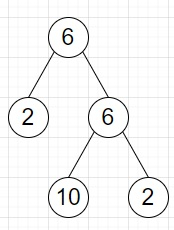# Program to check whether inorder sequence of a tree is palindrome or not in Python

Suppose we have a binary tree where each node contains a digit from 0-9, we have to check whether its in-order traversal is palindrome or not.

So, if the input is likethen the output will be True, as its inorder traversal is [2,6,10,6,2].

To solve this, we will follow these steps −

• if root is null, then
• return True
• stack := a new stack
• curr := root
• inorder := a new list
• while stack is not empty or curr is not null, do
• while curr is not null, do
• push curr into stack
• curr := left of curr
• node := popped element from stack
• insert value of node at the end of inorder
• curr := right of node
• return true when inorder is same as inorder in reverse order, otherwise false.

Let us see the following implementation to get better understanding −

## Example

Live Demo

class TreeNode:
def __init__(self, data, left = None, right = None):
self.val = data
self.left = left
self.right = right
class Solution:
def solve(self, root):
if not root:
return True
stack = []
curr = root
inorder = []
while stack or curr:
while curr:
stack.append(curr)
curr = curr.left
node = stack.pop() inorder.append(node.val)
curr = node.right
return inorder == inorder[::-1]
ob = Solution()
root = TreeNode(6)
root.left = TreeNode(2)
root.right = TreeNode(6)
root.right.left = TreeNode(10)
root.right.right = TreeNode(2)
print(ob.solve(root))

## Input

root = TreeNode(6)
root.left = TreeNode(2)
root.right = TreeNode(6)
root.right.left = TreeNode(10)
root.right.right = TreeNode(2)

## Output

True